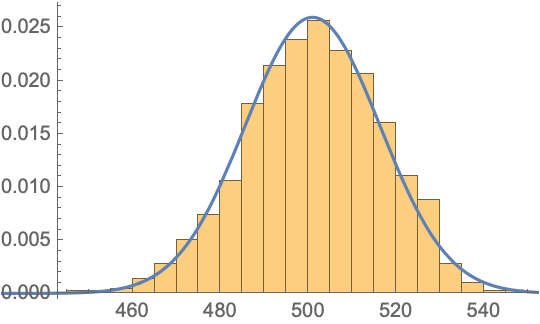#Function Repository Resource:

# BinaryRunRandomnessTest

Conduct a runs–based test on a sequence of zeros and ones

Contributed by: Emmy/Noah Blumenthal
noahb320@gmail.com
emmyb320@bu.edu
 ResourceFunction["BinaryRunRandomnessTest"][sequence] conducts a runs–based randomness test on a sequence of zeros and ones and returns an associated p-value. ResourceFunction["BinaryRunRandomnessTest"][sequence,"properties"] conducts a runs–based randomness test on a sequence of zeros and ones and returns an associated property.

## Details and Options

Properties include:
 "TestStatistic" returns the test statistic "PValue" returns the p-value associated with the test
The test statistic is generated by counting the total number of runs of both zeros and ones.
The test works only for sequences of zeros and ones.
Results are generally invalid for sequence lengths short than 100.

## Examples

### Basic Examples

Generate a sequence of random integers and apply a runs-based test:

 In:=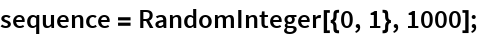Visualize the sequence:

 In:=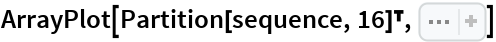Out=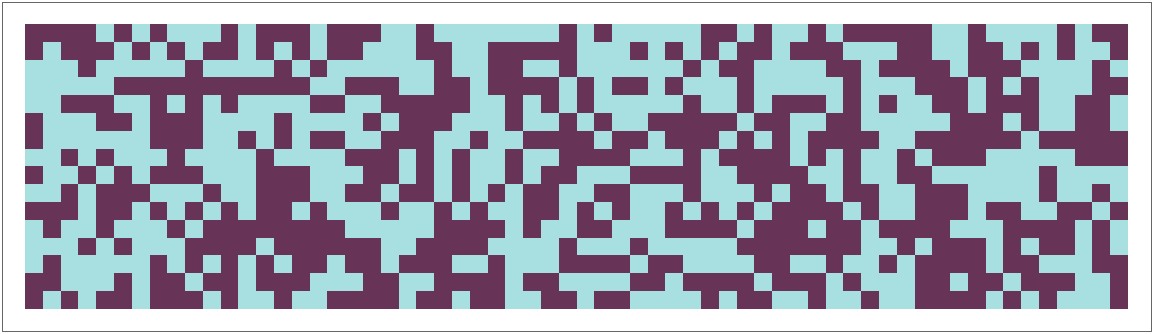In:=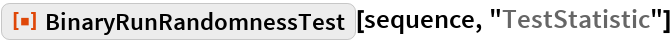Out=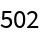In:=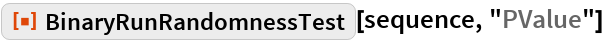Out=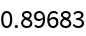### Applications

Reject a non-random sequence:

 In:=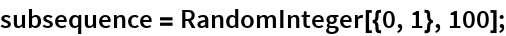In:=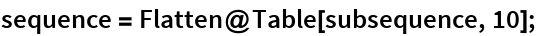In:=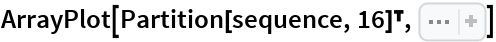Out=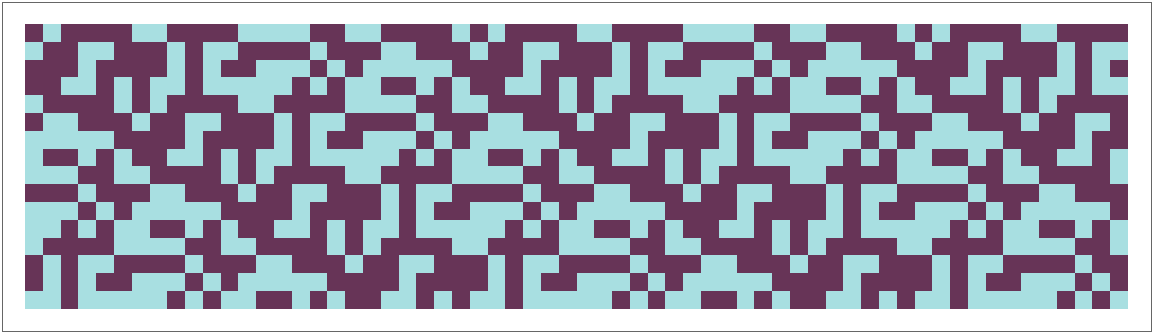In:=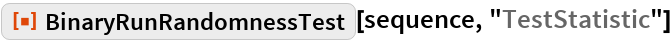Out=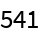In:=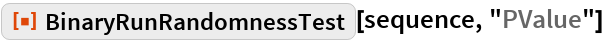Out=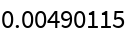Test the randomness of rule 30:

 In:=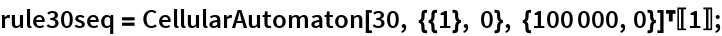In:=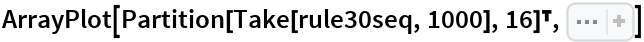Out=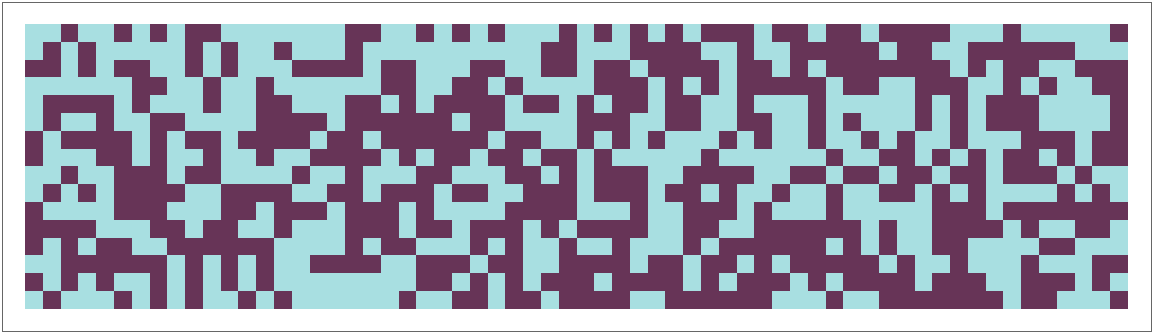In:=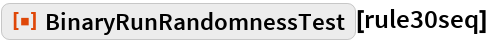Out=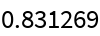### Possible Issues

BinaryRunRandomnessTest requires sequences of length 100 or more:

 In:=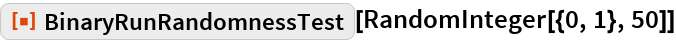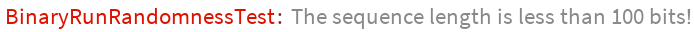### Neat Examples

Visualize the sampling distribution of the test statistic:

 In:=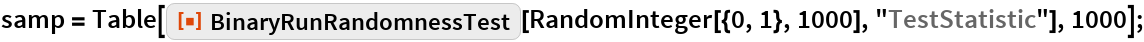In:=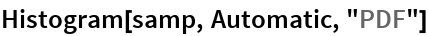Out=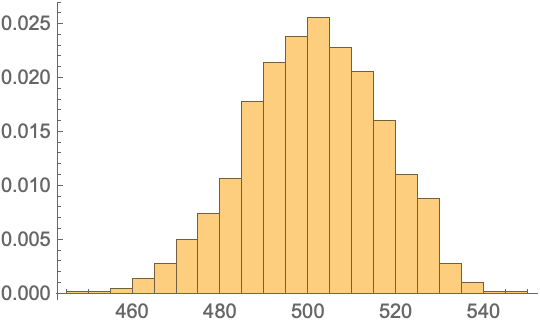In:=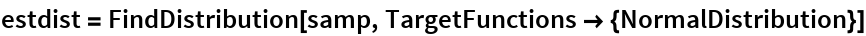Out=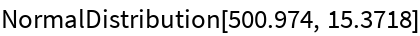In:=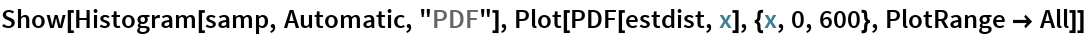Out=